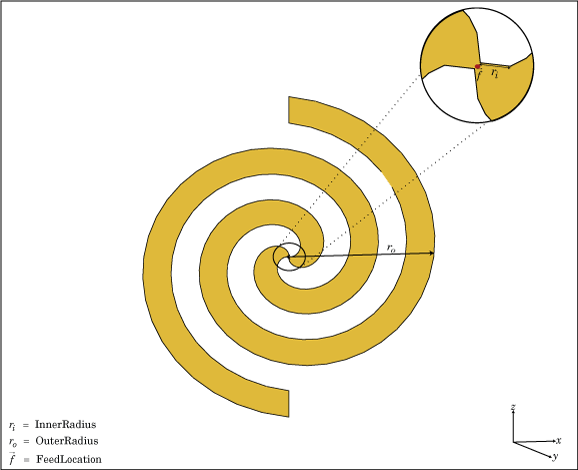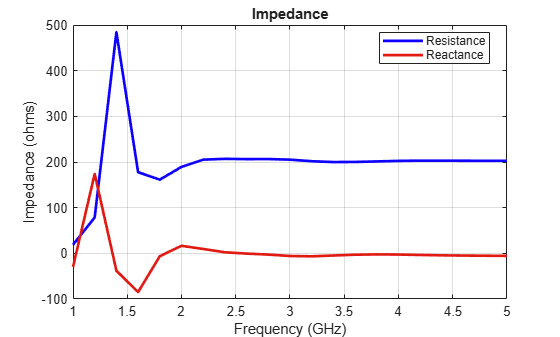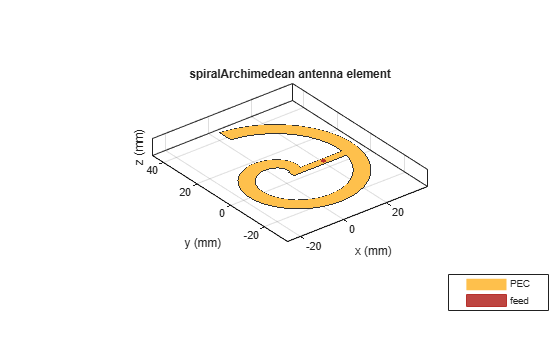# spiralArchimedean

Create Archimedean spiral antenna

## Description

The `spiralArchimedean` object creates a planar Archimedean spiral antenna on the xy- plane. The default Archimedean spiral is always center fed and has two arms. The field characteristics of this antenna are frequency independent. A realizable spiral has finite limits on the feeding region and the outermost point of any arm of the spiral. The spiral antenna exhibits a broadband behavior. The outer radius imposes the low frequency limit and the inner radius imposes the high frequency limit. The arm radius grows linearly as a function of the winding angle.

The equation of the Archimedean spiral is:

`$r={r}_{0}+a\varphi$`

where:

• r0 is the inner radius

• a is the growth rate

• ϕ is the winding angle of the spiral

Archimedean spiral antenna is a self-complementary structure, where the spacing between the arms and the width of the arms are equal. The default antenna is center fed. The feed point coincides with the origin. The origin is in the xy- plane.## Creation

### Syntax

``ant = spiralArchimedean``
``ant = spiralArchimedean(Name,Value)``

### Description

``` `ant = spiralArchimedean` creates a planar Archimedean spiral on the X-Y plane. By default, the antenna operates over a broadband frequency range of 3–5 GHz.```

example

``` `ant = spiralArchimedean(Name,Value)` sets properties using one or more name-value pairs. For example, `ant = spiralArchimedean('Turns',6.25)` creates a Archimedean spiral of 6.25 turns.```

### Output Arguments

expand all

MATLAB object, returned as scalar `spiralArchimedean` object.

## Properties

expand all

Number of arms, specified as a scalar integer. You can also create a single arm Archimedean spiral by specifying `NumArms` is equal to one.

Example: `'NumArms',1`

Example: `ant.NumArms = 1`

Data Types: `double`

Number of turns of the spiral antenna, specified as a scalar.

Example: `'Turns',2`

Example: `ant.Turns = 2`

Data Types: `double`

inner radius of the spiral antenna, specified as a scalar in meters.

Example: `'InnerRadius',1e-3`

Example: `ant.InnerRadius = 1e-3`

Data Types: `double`

Outer radius of the spiral antenna, specified as a scalar in meters.

Example: `'OuterRadius',1e-3`

Example: `ant.OuterRadius = 1e-3`

Data Types: `double`

Direction of the spiral turns (windings), specified as `'CW'` or `'CCW'`.

Example: `'WindingDirection'`,`'CW'`

Example: `ant.WindingDirection = CW`

Data Types: `char` | `string`

Type of the metal used as a conductor, specified as a metal material object. You can choose any metal from the `MetalCatalog` or specify a metal of your choice. For more information, see `metal`. For more information on metal conductor meshing, see Meshing.

Example: ```m = metal('Copper'); 'Conductor',m```

Example: ```m = metal('Copper'); ant.Conductor = m```

Lumped elements added to the spiral antenna feed, specified as a lumped element object. You can add a load anywhere on the surface of the antenna. By default, it is at the origin. For more information, see `lumpedElement`.

Example: `'Load',lumpedelement`. `lumpedelement` is the object for the load created using `lumpedElement`.

Example: ```ant.Load = lumpedElement('Impedance',75)```

Data Types: `double`

Tilt angle of the antenna, specified as a scalar or vector with each element unit in degrees. For more information, see Rotate Antennas and Arrays.

Example: `Tilt=90`

Example: `Tilt=[90 90]`,`TiltAxis=[0 1 0;0 1 1]` tilts the antenna at 90 degrees about the two axes defined by the vectors.

Note

The `wireStack` antenna object only accepts the dot method to change its properties.

Data Types: `double`

Tilt axis of the antenna, specified as:

• Three-element vector of Cartesian coordinates in meters. In this case, each coordinate in the vector starts at the origin and lies along the specified points on the X-, Y-, and Z-axes.

• Two points in space, each specified as three-element vectors of Cartesian coordinates. In this case, the antenna rotates around the line joining the two points in space.

• A string input describing simple rotations around one of the principal axes, 'X', 'Y', or 'Z'.

Example: `TiltAxis=[0 1 0]`

Example: `TiltAxis=[0 0 0;0 1 0]`

Example: `TiltAxis = 'Z'`

Data Types: `double`

## Object Functions

 `show` Display antenna, array structures or shapes `info` Display information about antenna or array `axialRatio` Axial ratio of antenna `beamwidth` Beamwidth of antenna `charge` Charge distribution on antenna or array surface `current` Current distribution on antenna or array surface `design` Design prototype antenna or arrays for resonance around specified frequency `efficiency` Radiation efficiency of antenna `EHfields` Electric and magnetic fields of antennas; Embedded electric and magnetic fields of antenna element in arrays `impedance` Input impedance of antenna; scan impedance of array `mesh` Mesh properties of metal, dielectric antenna, or array structure `meshconfig` Change mesh mode of antenna structure `optimize` Optimize antenna or array using SADEA optimizer `pattern` Radiation pattern and phase of antenna or array; Embedded pattern of antenna element in array `patternAzimuth` Azimuth pattern of antenna or array `patternElevation` Elevation pattern of antenna or array `rcs` Calculate and plot radar cross section (RCS) of platform, antenna, or array `returnLoss` Return loss of antenna; scan return loss of array `sparameters` Calculate S-parameter for antenna and antenna array objects `vswr` Voltage standing wave ratio of antenna

## Examples

collapse all

Create and view a 2-turn Archimedean spiral antenna with a 1 mm starting radius and 40 mm outer radius.

```sa = spiralArchimedean('Turns',2, 'InnerRadius',1e-3, 'OuterRadius',40e-3); show(sa)```Calculate the impedance of an Archimedean spiral antenna over a frequency range of 1-5 GHz.

```sa = spiralArchimedean('Turns',2, 'InnerRadius',1e-3, 'OuterRadius',40e-3); impedance(sa, linspace(1e9,5e9,21));```Create and view a single-arm Archimedean spiral.

```ant = spiralArchimedean; ant.NumArms = 1```
```ant = spiralArchimedean with properties: NumArms: 1 Turns: 1.5000 InnerRadius: 5.0000e-04 OuterRadius: 0.0398 WindingDirection: 'CCW' Conductor: [1x1 metal] Tilt: 0 TiltAxis: [1 0 0] Load: [1x1 lumpedElement] ```
`show(ant)`Balanis, C.A. Antenna Theory. Analysis and Design, 3rd Ed. New York: Wiley, 2005.

 Nakano, H., Oyanagi, H. and Yamauchi, J. “A Wideband Circularly Polarized Conical Beam From a Two-Arm Spiral Antenna Excited in Phase”. IEEE Transactions on Antennas and Propagation. Vol. 59, No. 10, Oct 2011, pp. 3518-3525.

 Volakis, John. Antenna Engineering Handbook, 4th Ed. McGraw-Hill